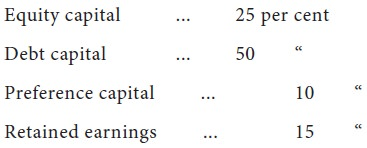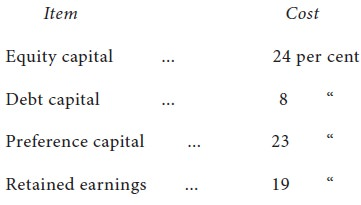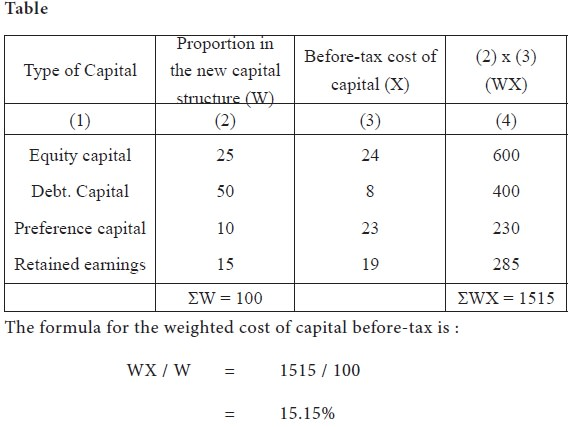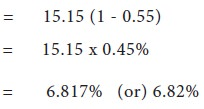Home | ARTS | Financial Management | Weighted Average Cost of Capital

# Weighted Average Cost of Capital

Posted On :  20.06.2018 01:28 am

Cost of capital does not refer to the cost of some specific source in the financial decision-making.

Weighted Average Cost of Capital

Cost of capital does not refer to the cost of some specific source in the financial decision-making. It should be the over- all cost of all sources and we should consider the weighted cost of capital. Weights are given in proportion to each source of funds in the capital structure; then weighted average cost of capital is calculated.

For calculating the weighted average cost of capital, we should know the capital structure of the company. Let us assume that the proposed capital structure of a company after new financing would be as follows:Secondly, we should calculate the cost of different types of capital stated above, before-tax in the manner in which we studied so far.

Suppose the firm has calculated the cost of each source of capital before-tax as follows:with these figures, the weighted average cost of capital is calculated for the company as shown in the following Table.The weighted average cost of capital in the above imaginary illustration is 15.12 per cent, before-tax.

After-tax cost of capital = Before-tax cost (1 — tax-rate). Assuming the tax-rate as 55% after-tax cost of capital comes to :This average cost of capital provides us a measure of the minimum rate of return which the proposed investment must earn to turn out to be acceptable.

All business decisions relating to capital budgeting and assessment of cost of capital are made under conditions of uncertainty. The management cannot ignore the risks and uncertainties associated with capital budgeting. Capital budgeting is influenced by many factors like the industrial policy of the government, location pattern, and Government’s policy on investments, benefits of tax incentives and the availability of inputs.
Tags : Financial Management - Capital Budgeting – A Conceptual Framework
Last 30 days 117 views